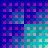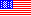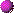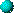#\$BJF_78&5f<<\$N3X0LO@J8(BIn English

[ ] \$BFb\$N?t;z\$OG/EY\$rI=\$7\$F\$\$\$^\$9!%(B

## \$BGn;NO@J8(B• Writing an Operating System with a Strictly Typed Assembly Language
\$B87L)\$K7?IU\$1\$5\$l\$?%"%;%s%V%j8@8l\$rMQ\$\$\$?%*%Z%l!<%F%#%s%0%7%9%F%`\$N5-=R(B
(\$BA0ED(B \$B=S9T(B)
• Middleware Systems for Enabling users to Adapt to Dynamic Changes in Execution Environments
\$B (\$B6bED(B \$B7{Fs(B)• Implementation of a Fail-Safe ANSI C Compiler
\$B0BA4\$J(B ANSI C \$B%3%s%Q%\$%i\$N (\$BBg4d(B \$B42(B)
• Defending against Distributed Denial of Service Attacks
\$BJ,;6%5!<%S%9Dd;_967b\$NBP:vJ}<0(B (Eric Y. Chen)
• Verification of Concurrent Programs using Proof Assistants
\$BDjM}>ZL@4o\$K4p\$E\$/JB9T%W%m%0%i%`\$N8!>Z(B (Reynald Affeldt)• Scalable Dynamic Memory Management Module on Shared Memory Multiprocessors
\$B6&M-%a%b%jJBNs7W;;5!>e\$N%9%1!<%i%V%k\$JF0E*%a%b%j4IM}%b%8%e!<%k(B
(\$B1sF#(B \$BIRIW(B)• Achieving High Performance for Parallel Programs that Contain Unscalable Modules
\$B%9%1!<%i%V%k\$G\$J\$\$%b%8%e!<%k\$r4^\$`JBNs%W%m%0%i%`\$K\$*\$1\$k9b@-G=\$NC#@.(B
(\$BBg;3(B \$B7C90(B)
• Regular Expression Types for XML
XML\$B\$N\$?\$a\$N@55,I=8=7?(B
(\$B:YC+(B \$B@2IW(B)• Analyses and Reduction of Operational Overhead in Computer-Assisted Drawing:
\$B7W;;5!;Y1g\$NIA2h\$K\$*\$1\$kA`:nIi2Y\$NJ,@O\$H:o8:(B
(\$B2OFbC+(B \$B9,;R(B)
• Architecture Design and Compilation Techniques Using Partial Evaluation in Reflective Concurrent Object-Oriented Languages:
\$BJB9T%*%V%8%'%/%H;X8~8@8l\$K\$*\$1\$k<+8JH?1G%"!<%-%F%/%A%c\$N@_7W\$H(B \$BItJ,7W;;\$rMQ\$\$\$?%3%s%Q%\$%kJ}K!(B
(\$BA}86(B \$B1QI'(B)• Theoretical Properties and Efficient Satisfaction of Hierarchical Constraint Systems:
\$B3,AX@)Ls7O\$NM}O@E*@-CK!(B
(\$B:YIt(B \$BGn;K(B)
• Efficient and Reusable Implementation of Fine-Grain Multithreading and Garbage Collection on Distributed-Memory Parallel Computers:
\$BJ,;65-21JBNs7W;;5!\$N\$?\$a\$N8zN(E*\$G:FMxMQ2DG=\$J(B \$B:YN3EY%^%k%A%9%l%C%G%#%s%05Z\$S%4%_=8\$a(B
(\$BED1:(B \$B7r)• A Concurrent Object-Oriented Programming Language System for Highly Parallel Data-Driven Computers and its Applications.
(\$BH,?y(B \$B>;9((B)Postscript file: A4 size, letter size (590k bytes each)
• Language Features for Extensibility and Re-use in Concurrent Object-Oriented Languages.
(\$B>>2,(B \$BAo(B)Postscript file: A4 size, letter size (923k bytes each)• A Reflective Object-Oriented Concurrent Language for Distributed Environments:
\$BJ,;64D6-\$K\$*\$1\$k%j%U%l%/%F%#%V\$JJBNs%*%V%8%'%/%H;X8~8@8l(B
(\$B0l?y(B \$BM5;V(B)

## \$B=\$;NO@J8(B• Combining Type-Based Analysis and Model Checking for Finding Counterexamples against Non-Interference
\$B7?2r@O\$H%b%G%k8!::\$rAH\$_9g\$o\$;\$?Hs43>D@-\$NH?NcH/8+K!(B
(\$B3\$Ln(B \$B9-;V(B)
• Continuation Join Point
\$B7QB3%8%g%\$%s%]%\$%s%H(B
(\$B1sF#(B \$BPR2p(B)
• Application-Specific Virtual Environments for Migration and Checkpointing
\$B%"%W%j%1!<%7%g%s\$KFC2=\$7\$?7W;;0\F0\$d%A%'%C%/%]%\$%s%F%#%s%0\$N\$?\$a\$N2>A[4D6-(B
(\$B2#;3(B \$BM[2p(B)
• A Framework Using a Common Language to Build Program Verifiers for Low-Level Languages
\$BDc5i8@8l\$N%W%m%0%i%`8!>Z4o\$r9=@.\$9\$k\$?\$a\$N6&DL8@8l\$rMQ\$\$\$?%U%l!<%`%o!<%/(B
(\$B5HLn(B \$B)• Model Checking for Computer Game Scenarios
\$B%b%G%k8!::M}O@\$N%2!<%`%7%J%j%*\$X\$NE,MQ(B
(\$B@6LZ(B \$B>;(B)
• Region-Based Memory Management for Dynamic Typed Language
\$BF0E*7?IU\$-8@8l\$N\$?\$a\$N%j!<%8%g%s?dO@\$K4p\$E\$/%a%b%j4IM}(B
(\$B1JED(B \$B>O?M(B)
• Designing and Implementing a Distributed Component Language with Transparent Mobility
\$BF)L@\$J0\F0\$,2DG=\$JJ,;6%3%s%]!<%M%s%H8@8l\$N@_7W\$H (\$BA};3(B \$BN4(B)• Implementing a Runtime System for Parallel and Distributed Computation on the Internet and Its Applications
\$B%\$%s%?!<%M%C%H>e\$NJBNsJ,;67W;;\$N\$?\$a\$N (\$B6bED(B \$B7{Fs(B)
• An Efficient Garbage Collector in the Presence of Ambiguous References
\$B[#Kf\$J%]%\$%s%?\$NB8:_2<\$G\$N8zN(E*\$J%4%_=8\$a(B
(\$B>.NS(B \$B5AFA(B)
• Regular Expression Types for Strings in a Text Processing Language
\$B%F%-%9%H=hM}8@8l\$K\$*\$1\$kJ8;zNs\$N\$?\$a\$N@55,I=8=7?(B
(\$BEDJ%(B \$BD>(B)
• Java Bytecode Transformation for Fine Grain CPU Resource Management
Java\$B%P%\$%H%3!<%IJQ49\$K\$h\$k:YN3EY(BCPU\$B;q8;4IM}(B
(\$BB.?e(B \$BM:B@(B)
• Resource Usage Analysis with References
\$B;2>H\$r07\$C\$?;q8;;HMQ2r@O(B
(\$B?e>e(B \$BC#IW(B)• Supporting Object-Oriented Features in Run-time Bytecode Specialization
\$B (\$B%l%J%k%I!&%"%U%'%k%H(B)
• A Self-organizing Hierarchical Network for Global Parallel Computing
\$B9-0hJ,;6JBNs7W;;\$N\$?\$a\$N<+8JJT@.2DG=\$J3,AXE*%M%C%H%o!<%/(B
(\$B>eED(B \$BM[J?(B)
• Dynamic Thread Mapping for Distributed Data Intensive Applications
\$BJ,;6%G!<%?(Bintensive\$B\$J%"%W%j%1!<%7%g%s\$N\$?\$a\$NF0E*%9%l%C%IG[CV(B
(\$B:dK\(B \$B?rM5(B)
• A Type System for Object Usage Analysis in Java Bytecode
Java\$B%P%\$%H%3!<%I\$K\$*\$1\$k%*%V%8%'%/%H\$N;HMQ2r@O\$N\$?\$a\$N7?%7%9%F%`(B
(\$BIMCf(B \$B?.9T(B)
• Distributed web crawling with dynamically joining/leaving resources
\$BF0E*\$KA}8:\$9\$k;q8;\$rMxMQ\$7\$?9-0hJ,;6(B web crawling
(\$B9?(B \$B=_>M(B)
• Safe Execution of User Programs in Kernel Mode using Typed Assembly Language
\$B7?IU\$-%"%;%s%V%j8@8l\$rMQ\$\$\$F%f!<%6%W%m%0%i%`\$r%+!<%M%k%b!<%I\$G (\$BA0ED(B \$B=S9T(B)
• Designing a Virtual Machine Independent Compiler Interface
\$B2>A[%^%7%sFHN)\$J%3%s%Q%\$%i%\$%s%?%U%'!<%9\$N@_7W(B
(\$B;3Fb(B \$BM5;K(B)• Dynamic accesss control of mobile objects by switching name spaces
\$BL>A06u4V\$N@Z\$jBX\$(\$K\$h\$k0\F0%*%V%8%'%/%H\$NF0E*%"%/%;%9@)8f(B
(\$B:#0f(B \$B7rCK(B)
• Lazy Task Creation with Stack Swapping to Improve Memory Locality
\$B%a%b%j6I=j@-\$r9b\$a\$k%9%?%C%/8r49J}<0\$rF3F~\$7\$?CY1d%?%9%/@8@.(B
(\$B30;3(B \$B=c@8(B)
• An extension of C++ that supports thread migration with little loss of normal execution efficiency
\$BDL>o\$N (\$BB?2l(B \$BF`M3B@(B)• Software-based Fine-grained Memory Protection Supporting Dynamic Changes of Attributes:
\$BJ]8nB0@-\$NF0E*JQ99\$,2DG=\$J%=%U%H%&%'%"\$K\$h\$k:YN3EY%a%b%j\$NJ]8n(B
(\$B8eF#(B \$BNi;K(B)
• Lazy Task Creation on Shared Memory Machines for C Programs:
(\$BEDC<(B \$BK.CK(B)
• Research on Effects of Mobile Object Programming with Describing Distributed Application:
\$B0\F0%*%V%8%'%/%H%W%m%0%i%_%s%0\$NJ,;6=hM}5-=R\$K\$h\$kM-8z@-\$N8&5f(B
(\$B?M8+(B \$B=SB@O:(B)
• Implementation of an Efficient and Reusable Distributed Garbage Collector and Evaluation of Its Performance:
\$B8zN(E*\$G:FMxMQ2DG=\$JJ,;6(BGC\$B%i%\$%V%i%j\$N@_7W\$H@-G=I>2A(B
(\$B;3K\(B \$BBY1'(B)• A Scalable Mark-Sweep Garbage Collector on Large-Scale Shared-Memory Machines
\$BBg5,LO6&M-%a%b%j%^%7%s>e\$N%9%1!<%i%V%k\$J%^!<%/%9%\$!<%WK!%,!<%Y%8%3%l%/%?(B
(\$B1sF#(B \$BIRIW(B)
• An Efficient Compilation Framework for Parallel Programming Languages Based on a Concurrent Process Calculus
\$BJB9T7W;;\$K4p\$E\$/JBNs%W%m%0%i%_%s%08@8l\$N\$?\$a\$N8zN(E*\$J%3%s%Q%\$%k\$NOHAH\$_(B
(\$BBg;3(B \$B7C90(B)• A Study on Debugging Schemes in Concurrent Programs on Massively Parallel Processors:
\$BD6JBNs7W;;5!>e\$NJBNs%W%m%0%i%`MQ%G%P%C%0J}<0\$N8&5f(B
• An Extension to a Parallel Constraint Logic Programming Language For Applications in Optimization Problems:
\$B:GE,2=LdBj\$X\$N1~MQ\$N\$?\$a\$NJBNs@)LsO@M}7?8@8l\$N3HD%(B
(\$B:#Ln(B \$BOB9@(B)
• A Study on Structure of Concurrent Object-Oriented Languages:
\$BJBNs%*%V%8%'%/%H;X8~8@8l\$N9=B\$\$K4X\$9\$k8&5f(B
(\$B4X8}(B \$BN6O:(B)
• Efficient Satisfaction of Constraint Hierarchies:
\$B@)Ls3,AX\$N8zN(E*\$J2r>CK!(B
(\$B:YIt(B \$BGn;K(B)• Design and Implementation of Concurrent Object-Oriented Programming Languages on Stock Multicomputers:
\$BJBNs%*%V%8%'%/%H;X8~8@8l\$N%^%k%A%3%s%T%e!<%?>e\$K\$*\$1\$k@_7W\$*\$h\$S (\$BED1:(B \$B7r)Postscript file:
A4 size, letter size (375k bytes each)
• Study on a Reflective Architecture to Provide Efficient Dynamic Resource Management for Highly-Parallel Object-Oriented Applications:
\$B9bJBNs%*%V%8%'%/%H;X8~%"%W%j%1!<%7%g%s\$N\$?\$a\$N8zN(\$N\$h\$\$F0E*;q8;4IM}J}<0\$r(B \$BDs6!\$9\$k<+8JH?1G%"!<%-%F%/%A%c\$N8&5f(B
(\$BA}86(B \$B1QI'(B)Postscript file: A4 size, letter size (278k bytes each)
• Lexicon Acquisition in HPSG-based Grammars:
(\$BD;_7(B \$B7rB@O:(B)
• An Application of Parallel Object-Oriented Methodology to Molecular Dynamics Simulation
(Naohito Omori)
• Compiler Design and Implementation Techniques for Parallel Constraint Logic Programming Languages:
\$BJBNs@)LsO@M}7?8@8l\$N%3%s%Q%\$%i@_7W\$H (\$BD9DM(B \$B2mL@(B)
• Interactive Generation of Graphical User Interfaces by Multiple Visual Examples:
\$BJ#?t\$N;k3PE*Nc\$K\$h\$kD>@\A`:n%\$%s%?!<%U%'!<%9\$NBPOCE* (\$B5\2<(B \$B7r(B)• Model Checking of Finite State CSP:
\$BM-8B>uBV(B CSP \$B\$N%b%G%k8!::(B
(\$B@u0f(B \$B7r0l(B)
• A Framework for Describing Mental States and their Dynamic Changes of Dialog Participants:
\$BBPOC;22C (\$BB

## \$B3X;NO@J8(B• A Library for Replay-based Recovery
\$B%j%W%l%\$\$K4p\$E\$/%j%+%P%j\$N\$?\$a\$N%i%\$%V%i%j(B
(\$BBg=;(B \$BM5G7(B)
• Implementation of Programming Language based on Alias Types and Measurement of Its Ability
Alias Types \$B\$K4p\$E\$/%W%m%0%i%_%s%08@8l\$N2A(B
(\$B:4F#(B \$B=U4z(B)
• Implementation of Information Flow Analysis using Dynamic Type Checking
\$BF0E*\$J7?8!::\$rMQ\$\$\$?>pJsN.2r@O\$N (\$B;32<(B \$BNJB"(B)• Effective Software Obfuscation by Mixing Instructions and Data
\$BL?Na\$H%G!<%?\$N:.9g\$K\$h\$k8z2LE*\$J%=%U%H%&%'%"\$NFqFI2=(B
(\$B:4F#(B \$B=(L@(B)
• Detecting Intrusions on Windows Operating Systems by Monitoring System Services
System Service \$B\$N4F;k\$K\$h\$k(B Windows \$BMQ?/F~8!CN%7%9%F%`(B
(\$BEgK\(B \$BBgJe(B)
• Reliable Peer-to-Peer Framework for Massively Multiplayer Online Games
\$BBg5,LOB??M?t%*%s%i%\$%s%2!<%`\$N\$?\$a\$N?.Mj@-\$N\$"\$k(B Peer-to-Peer \$B\$NOHAH\$_(B
(\$B;3:j(B \$B9'M5(B)• Aspect-Oriented Virtual Machine for Supporting Dynamic Weaving and High-Level Features
\$B9b5i\$J8@8l5!G=\$r;}\$A%"%9%Z%/%H\$rF0E*\$KE,MQ\$9\$k2>A[5!3#(B
(\$B1sF#(B \$BPR2p(B)
• Region Representation Inference for Dynamically-Typed Language
\$BF0E*7?IU\$18@8l\$N0Y\$N%j!<%8%g%sI=8=?dO@(B
(\$B@nCf(B \$B??Lm(B)
• A Toolkit for Developing Extensible and Portable Checkpoint Systems
\$B3HD%@-\$H8_49@-\$r;}\$D%A%'%C%/%]%\$%s%H%7%9%F%`\$N9=C[\$rMF0W\$K\$9\$kJ}K!(B
(\$B2#;3(B \$BM[2p(B)
• Design and Implementation of a Self-Repairing Reference Monitor
\$B<+8J=\$I|7?%j%U%!%l%s%9%b%K%?\$N@_7W\$H (\$B5HLn(B \$B)• Parallelizing Programs Using Access Traces
\$B%"%/%;%9MzNr\$K\$h\$k%W%m%0%i%`\$NJBNs2=(B
(\$B%0%'%s(B \$B%t%'%H(B \$B%O(B)
• A Scenario Description Language Based on a State Transition Model and its Automatic Consistency Checking
\$B>uBVA+0\%b%G%k\$K4p\$E\$\$\$?%7%J%j%*5-=R8@8l\$N@_7W\$HF18@8l>e\$G\$N<+F08!>Z(B
(\$B@6LZ(B \$B>;(B)
• Soft Typing\$B\$rMxMQ\$7\$?(BScheme-to-Java\$B%H%i%s%9%l!<%?(B
Scheme-to-Java Translator with Soft Typing
(\$B1JED(B \$B>O?M(B)
• An Approach to Separate compilation of C++ Templates
C++\$B\$N%F%s%W%l!<%H4X?t\$rJ,3d%3%s%Q%\$%k2DG=\$K\$9\$k\$?\$a\$N%"%W%m!<%A(B
(\$BA};3(B \$BN4(B)• A New Type System for JVM Lock Primitive
JVM\$B\$K\$*\$1\$k%m%C%/\$N@09g@-8!>Z\$N\$?\$a\$N(B \$B?7\$7\$\$7?%7%9%F%`(B
(\$B4d4V(B \$BB@(B)
• Virtual Private Grid (VPG): A Command Shell for Utilizing Remote Machines Efficiently
Virtual Private Grid (VPG): \$B1s3V7W;;5!\$r8zN(E*\$KMxMQ\$9\$k%7%'%k(B
(\$B6bED(B \$B7{Fs(B)
• Java Bytecode Transformation for Fine Grain CPU Resource Management
Java\$B%P%\$%H%3!<%IJQ49\$K\$h\$k:YN3EY(BCPU\$B;q8;4IM}(B
(\$BB.?e(B \$BM:B@(B)
• Design and Implementation of Storage Server for Mobile Clients
\$B%b%P%\$%k%/%i%\$%"%s%H\$N\$?\$a\$N%9%H%l!<%8%5!<%P!<\$N%G%6%\$%s\$H (\$B?e>e(B \$BC#IW(B)• Single Memory Image for Heterogeneous Distributed Computing: Its Implementation and Evaluation
\$B0[2A(B
(\$B>eED(B \$BM[J?(B)
• Java Bytecode Translation for Transparent Migration
\$BF)L@\$J%^%\$%0%l!<%7%g%s\$N\$?\$a\$N(BJava\$B%P%\$%H%3!<%IJQ49(B
(\$B:dK\(B \$B?rM5(B)
• An Implementation of Distributed Cyclic Garbage Collection for Java RMI
Java RMI\$B\$G\$NJ,;64D>u%4%_=8\$a\$N (\$BIMCf(B \$B?.9T(B)
• Automatic Library Translation for Host-local Resources in Languages with Object Mobility
\$B%*%V%8%'%/%H0\F0@-\$r;}\$C\$?8@8l\$K\$*\$1\$k%[%9%H%m!<%+%k\$J%j%=!<%9\$N\$?\$a\$N<+F0%i%\$%V%i%jJQ49(B
(\$B;3Fb(B \$BM5;K(B)• Extending Java Virtual Machine to Improve Performance of Dynamically-Typed Languages:
\$BF0E*7?IU\$-8@8l\$N8zN(8~>e\$N\$?\$a\$N(BJava\$B2>A[%^%7%s\$N3HD%(B
(\$BBg4d(B \$B42(B)
(\$BBg3Q(B \$B<~J?(B)
• Copying Garbage Collection in the Presence of Uncertain Pointers:
\$BIT3N\$+\$J%]%\$%s%?\$NB8:_2<\$G\$N%3%T!<%\$%s%0%,!<%Y%8%3%l%/%7%g%s(B
(\$BEDCf(B \$B5A=c(B)• Implementation and Evaluation of Concurrent Object in Schematic:
Schematic\$B\$K\$*\$1\$kJBNs%*%V%8%'%/%H\$N2A(B
(\$B30;3(B \$B=c@8(B)
• Implementation of Mobile Scheme:
\$B%b!<%P%\$%k(BScheme\$B\$N (\$BB?2l(B \$BF`M3B@(B)• Improving execution efficiency of Tree-Structure-Based Parallel Programs:
\$BLZ9=B\$\$r07\$&JBNs%W%m%0%i%`\$N8zN(2=(B
(\$B8eF#(B \$BNi;K(B)
• Mobile Emacs Lisp: An Extension to Emacs Lisp for Efficient Editing on Wide-Area Network:
Mobile Emacs Lisp: \$B9-0h%M%C%H%o!<%/>e\$N8zN(E*\$JJT=8:n6H\$N\$?\$a\$N(BEmacs Lisp\$B\$N3HD%(B
(\$B?M8+(B \$B=SB@O:(B)
• A Research on Execution Performance of Parallel Programs on a Workstation Cluster:
\$BJBNs%W%m%0%i%`\$N%o!<%/%9%F!<%7%g%s%/%i%9%?>e\$K\$*\$1\$k (\$B;3K\(B \$BBY1'(B)• A Methodology for Constructing a Portable Garbage Collector on Parallel Machines:
\$BJBNs%^%7%s\$K\$*\$1\$k(BPortable\$B\$J(BGarbage Collector\$B\$N (\$B1sF#(B \$BIRIW(B)
• A General Framework for Compiling Fine-grain Threads in Concurrent Object-Oriented Languages:
\$BJBNs%*%V%8%'%/%H;X8~8@8l\$K\$*\$1\$k:YN3EY%9%l%C%I%3%s%Q%\$%k\$N\$?\$a\$N0lHLE*OHAH\$_(B
(\$BBg;3(B \$B7C90(B)• Study on Mechanisms For Multi-Object Synchronization and Their Implementation:
\$B%*%V%8%'%/%H4VF14|5!9=\$H\$=\$N (\$B8^==Mr(B \$B=_(B)
• Static Analysis on Communication for Asynchronous Concurrent Programming Languages:
\$BHsF14|JB9T8@8l\$K\$*\$1\$kDL?.\$N@EE*2r@O(B
(\$BCf=P(B \$B85
• Control Constructs in Concurrent Object-Oriented Languages:
\$BJBNs%*%V%8%'%/%H;X8~8@8l\$K\$*\$1\$k@)8f9=(B
(\$B:YC+(B \$B@2IW(B)
• Dialogue processing with Mental World Structure:
\$B?4E*@\$3&9=B\$\$rMQ\$\$\$?BPOC=hM}(B
(BEKKI Daisuke)
• Construction of a Japanese-text Generation System With Semantic-Head-Driven Generation:
\$B0UL# (\$B?9OF(B \$BIR(B)
• Automatic Acquisition of The Cost of Connectivity in A Japanese Morphological Analyzer:
\$BF|K\8l7ABVAG2r@O%7%9%F%`\$K\$*\$1\$kO"@\%3%9%H\$N<+F03MF@(B
(\$BLnH*(B \$B<~(B)• Type Inference for Polymorphic Reference with Subtyping:
\$BItJ,7?\$NF~\$C\$?B?Aj;2>H\$N\$?\$a\$N7??dO@(B
(\$BCfC+(B \$BL@90(B)
• A study on an implemenation of HACL compiler on multicomputers:
HACL\$B%3%s%Q%\$%i\$N%^%k%A%3%s%T%e!<%?>e\$G\$N (SHIMIZU Toshihiro)• An Efficient Implementation of a Parallel Constraint Logic Programming Language and its Applications:
\$BJBNs@)LsO@M}7?8@8l\$N8zN(E*\$J (\$B:#Ln(B \$BOB9@(B)
• An Algorithm of Distributed Garbage Collections on a Multicomputer and its Performance Evaluation:
\$BJBNs7W;;5!>e\$NJ,;6%,!<%Y!<%8!&%3%l%/%7%g%s\$N%"%k%4%j%:%`\$H\$=\$N@-G=I>2A(B
• A Constraint Solving Method for Real-Time Interaction in User Interfaces:
\$B%f!<%6%\$%s%?!<%U%'!<%9\$GCK!(B
(\$B:YIt(B \$BGn;K(B)
• An Object-Oriented Approach to Parsing for Unification-based Grammars:
\$B%*%V%8%'%/%H;X8~J}<0\$K\$h\$kC10l2=J82=\$rMQ\$\$\$?%Q!<%8%s%0(B
(Kengpang Lee)
• Construction of Type Inference Systems for Concurrent Object-Oriented Languages:
\$BJBNs%*%V%8%'%/%H;X8~8@8l\$N\$?\$a\$N7??dO@BN7O\$N9=C[(B
(\$B4X8}(B \$BN6O:(B)• Multilisp Implementation:
\$B%^%k%A%j%9%W\$N (\$B@u0f(B \$B7r0l(B)
• Fast Implementation of Production Systems:
\$B%W%m%@%/%7%g%s%7%9%F%`\$N9bB.2=(B